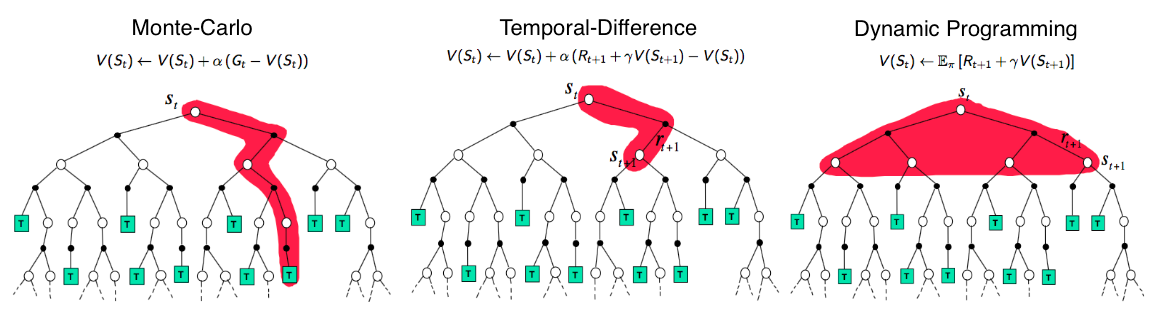# 介绍

Reinforcement learning is learning what to do ----how to map situations to actions ---- so as to maximize a numerical reward signal.

# 关键概念

RL 来自于人类学习的过程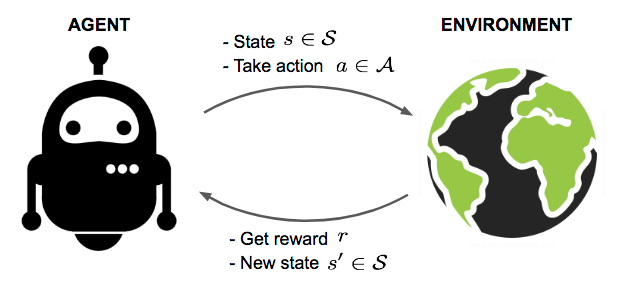• 已知模型: 基于模型的方法, 例如 DP, 可以用来求最优的解决方案
• 未知模型: 使用不完整的信息, 即 model-free 形式的 RL.

Reward Signal: 定义了 agent 学习的目标. agent 每次和环境交互, 环境返回一个 数值型(numerical) reward, 高速 agent 刚刚的 action 是好是坏, 可以理解为对 agent 的奖励或者惩罚. agent 与环境交流的序列/轨迹(episode/trajectory)为:

$$\tau=s_0,a_0,r_1,s_1,a_1,r_2,\cdots,s_{n-1},a_{n-1},r_n$$

$$s_{t+1}=f\left(s_{t}, a_{t}\right)$$

$$s_{t+1} \sim P\left(\cdot | s_{t}, a_{t}\right)$$

$$p(s_{t+1}|s_t,a_t,\cdots,s_0,a_0)=p(s_{t+1}|s_t,a_t)$$

\begin{aligned} p(\tau)&=p\left(s_{0}, a_{0}, s_{1}, a_{1}, \dots, s_{T-1}, a_{T}, s_T, r_T\right)\\ &=p(s_0)\prod_{t=0}^{T-1}\pi(a_t|s_t)p(s_{t+1}|s_t,a_t) \end{aligned}

## Model: 状态转移和回报

$$P(s', r \vert s, a) = \mathbb{P} [s_{t+1} = s', r_{t+1} = r \vert s_t = s, a_t = a]$$

$$P_{ss'}^a = P(s' \vert s, a) = \mathbb{P} [s_{t+1} = s' \vert s_t = s, a_t = a] = \sum_{r \in \mathcal{R}} P(s', r \vert s, a)$$

$$R(s, a) = \mathbb{E} [r_{t+1} \vert s_t = s, a_t = a] = \sum_{r\in\mathcal{R}} r \sum_{s' \in \mathcal{S}} P(s', r \vert s, a)$$

## 策略(Policy)

• 随机策略(stochastic policy): $\sum\pi(a|s)=1$
• 确定性策略(deterministic policy): $\pi(s)=P[a_t=a|s_t=t]:S\rightarrow{A}$.

$$\begin{array}{l}{a_{t}=\mu_{\theta}\left(s_{t}\right)} \\ {a_{t} \sim \pi_{\theta}\left(\cdot | s_{t}\right)}\end{array}$$

• 确定性策略: 一般用在连续行为空间中,
• 随机策略: 常用的两种随机策略: categoricaldiagonal Gaussian. 后者多用于连续空间. 这两种随机策略在行为的选择(sample from policy)和计算对数似然中有不同的计算方式. 在代码中, 他们都是使用 logits 作为输入的. 具体计算方式可见stochastic-policies

## Rewards 和 Return

reward 函数$R$用于产生参数立即回报:

$$r_t=R(s_t,a_t,s_{t+1})$$

1. 折扣回报(infinite-horizon discounted return): $R(\tau)=\sum_{t=0}^{\infty} \gamma^{t} r_{t}$适用于连续任务(即终止时间不定)
2. finite-horizon undiscounted return: $R(\tau)=\sum_{t=0}^{T} r_{t}$用于步长确定的任务.

$0\leq\gamma\leq{1}$是折扣率(discount rate), 它嗲表未来第$k$步的 reward 的价值只是在当前获得这个 reward 的 $\gamma^{k-1}$倍. 若$\gamma{<1}$, 上面的公式就会从在本发散的和变为前提为序列$\left\{r_k\right\}$的前提下转变为一个有限的值;若$\gamma=0$, agent变得目光短浅(myopic), 仅仅选择最大化$r_{t+1}$的动作;若$\gamma\rightarrow{1}$, 这个 agent 变得很"有远见". 这么做的目的:

• 未来的回报有很高的不确定性.
• 未来回报对现在没有即时的好处: 今天的幸福比五年后的幸福要好
• 求和会收敛.
• 不用担心状态转换图中的无限循环.

$$G(\tau)=\sum_{t=0}^{T-1} \gamma^{t} r_{t+1}$$

## 值函数(Value Function)

1. 定义$\pi$的On-Policy Value Function $v_\pi$ 表示以从$t$开始的以$s$为起始状态的 return 的期望:

$$\begin{equation} V^{\pi}(s)=\underset{\tau \sim \pi}{\mathrm{E}}\left[R(\tau) | s_{0}=s\right] \label{v_func} \end{equation}$$

1. 定义$\pi$的On-Policy Action-Value Function, $Q_\pi(s,a)$, 这里的$a$可能并不是根据策略$\pi$产生的, 但是接下来永远基于策略$\pi$:

$$\begin{equation} Q^{\pi}(s, a)=\underset{\tau \sim \pi}{\mathrm{E}}\left[R(\tau) | s_{0}=s, a_{0}=a\right] \label{q_func} \end{equation}$$

1. 最优的值函数(Optimal Value Function)以最大化 return 为目标:

$$V^{*}(s)=\max _{\pi} \underset{\tau \sim \pi}{\mathrm{E}}\left[R(\tau) | s_{0}=s\right]$$

1. 最优行为-值函数(Optimal Action-Value Function): 以$s$为起始状态采取一个任意的行为$a$并永远执行最优策略:

$$Q^{*}(s, a)=\max _{\pi} \underset{\tau \sim \pi}{\mathrm{E}}\left[R(\tau) | s_{0}=s, a_{0}=a\right]$$
$Q_\pi$和$V_\pi$之间的差值就是 advantage function(A值):$$A_{\pi}(s, a) = Q_{\pi}(s, a) - V_{\pi}(s)$$

$$V^{\pi}(s)=\underset{a \sim \pi}{E}\left[Q^{\pi}(s, a)\right]$$

$$V^{*}(s)=\max _{a} Q^{*}(s, a)$$

## The Optimal Q-Function and the Optimal Action

$$a^{*}(s)=\arg \max _{a} Q^{*}(s, a)$$

## 优化的目标

$$\begin{equation} \begin{split} \mathbb{E}_{\tau \sim p(\tau)}[G(\tau)]&=E_{s \sim p\left(s_{0}\right)}\left[\mathbb{E}_{\tau \sim p(\tau)}\left[\sum_{t=0}^{T-1} \gamma^{t} r_{t+1} | \tau_{s_{0}}=s\right]\right]\\ &=\mathbb{E}_{s \sim p\left(s_{0}\right)}\left[V^{\pi}(s)\right]\quad\text{带入}\eqref{v_func} \end{split} \label{obj_func} \end{equation}$$

# 马尔可夫决策过程(MDP)

agent 与环境在 MDP 交互过程: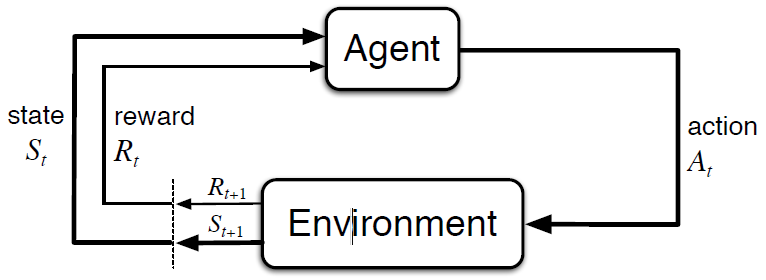• $\mathcal{S}$ 是有限的状态集
• $\mathcal{A}$ 是有限的动作集
• $P$ 是状态转移概率矩阵
• $R$ 是回报函数
• $\gamma$ 是折扣因子，用来对 return 进行折扣操作

# 贝尔曼方程(Bellman Equation)

V的离散的贝尔曼方程可以写为:

\begin{aligned} V^\pi(s) &= \mathbb{E}[R(\tau) \vert s_0 = s] \\ &=\mathbb{E}_{\tau_{0:T}\sim{p(\tau)}}[r_0+\gamma r_1+\gamma^2r_2+\cdots\vert \tau_{s_0} = s]\\ &=\mathbb{E}_{a\sim\pi(a|s)}\mathbb{E}_{s'\sim{p(s'|s,a)}}\mathbb{E}_{\tau_{1:T}\sim{p(\tau)}}[r(s_0,a,s')+\gamma\sum_{t=1}^{\infty}\gamma^{t-1}r_t\vert \tau_{s_1}=s']\\ &=\mathbb{E}_{a\sim\pi(a|s)}\mathbb{E}_{s'\sim{p(s'|s,a)}}\left[r(s,a,s')+\gamma\mathbb{E}_{\tau_{1:T}\sim{p(\tau)}}\left[\sum_{t=1}^\infty\gamma^{t-1}r_t\vert\tau_{s_1}=s'\right]\right]&\scriptstyle\text{;r为常数}\\ &=\mathbb{E}_{a\sim\pi(a|s)}\color{red}{\mathbb{E}_{s'\sim{p(s'|s,a)}}[r(s,a,s')+\gamma V^\pi(s')]} \end{aligned}

$$V^\pi(s)=\mathbb{E}_{a\sim\pi(a|s)}[Q^\pi(s,a)]$$

$$Q^\pi(s,a)=\mathbb{E}_{s'\sim{p(s'|s,a)}}\left[r(s,a,s')+\gamma\mathbb{E}_{a'\sim\pi(a'|s')}[Q^\pi(s',a')]\right]$$

## 贝尔曼期望方程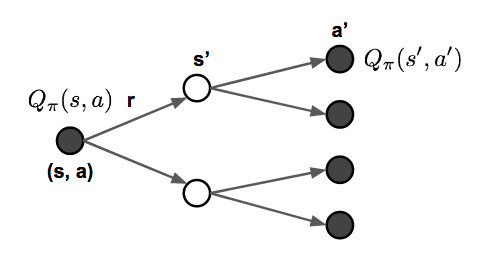\begin{aligned} V_{\pi}(s) &= \sum_{a \in \mathcal{A}} \pi(a \vert s) Q_{\pi}(s, a) \\ Q_{\pi}(s, a) &= R(s, a) + \gamma \sum_{s' \in \mathcal{S}} P_{ss'}^a V_{\pi} (s') \\ V_{\pi}(s) &= \sum_{a \in \mathcal{A}} \pi(a \vert s) \big( R(s, a) + \gamma \sum_{s' \in \mathcal{S}} P_{ss'}^a V_{\pi} (s') \big) \\ Q_{\pi}(s, a) &= R(s, a) + \gamma \sum_{s' \in \mathcal{S}} P_{ss'}^a \sum_{a' \in \mathcal{A}} \pi(a' \vert s') Q_{\pi} (s', a') \end{aligned}

## 贝尔曼最优方程

\begin{aligned} V_*(s) &= \max_{a \in \mathcal{A}} Q_*(s,a)\\ Q_*(s, a) &= R(s, a) + \gamma \sum_{s' \in \mathcal{S}} P_{ss'}^a V_*(s') \\ V_*(s) &= \max_{a \in \mathcal{A}} \big( R(s, a) + \gamma \sum_{s' \in \mathcal{S}} P_{ss'}^a V_*(s') \big) \\ Q_*(s, a) &= R(s, a) + \gamma \sum_{s' \in \mathcal{S}} P_{ss'}^a \max_{a' \in \mathcal{A}} Q_*(s', a') \end{aligned}

# 通用方法

## DP

### 策略评估(Policy Evaluation)

$$V_{t+1}(s) = \mathbb{E}_\pi [r + \gamma V_t(s) | S_t = s] = \sum_a \pi(a \vert s) \sum_{s', r} P(s', r \vert s, a) (r + \gamma V_k(s'))$$

### 策略改善(Policy Improvement)

$$Q_\pi(s, a) = \mathbb{E} [R_{t+1} + \gamma V_\pi(S_{t+1}) \vert S_t=s, A_t=a] = \sum_{s', r} P(s', r \vert s, a) (r + \gamma V_\pi(s'))$$

### 策略迭代(Policy Iteration)

$$\pi_0 \xrightarrow[]{\text{evaluation}} V_{\pi_0} \xrightarrow[]{\text{improve}} \pi_1 \xrightarrow[]{\text{evaluation}} V_{\pi_1} \xrightarrow[]{\text{improve}} \pi_2 \xrightarrow[]{\text{evaluation}} \dots \xrightarrow[]{\text{improve}} \pi_* \xrightarrow[]{\text{evaluation}} V_*$$

\begin{aligned} Q_\pi(s, \pi'(s)) &= Q_\pi(s, \arg\max_{a \in \mathcal{A}} Q_\pi(s, a)) \\ &= \max_{a \in \mathcal{A}} Q_\pi(s, a) \geq Q_\pi(s, \pi(s)) = V_\pi(s) \end{aligned}

## Monte-Carlo 方法

$$V(s) = \frac{\sum_{t=1}^T \mathbb{I}[S_t = s] G_t}{\sum_{t=1}^T \mathbb{I}[S_t = s]}$$

• every-visit 每次都记录每个 episode 中状态$s$
• first-visit 记录(record)在一次 episode 中状态$s$的第一次访问

$$Q(s, a) = \frac{\sum_{t=1}^T \mathbb{I}[S_t = s, A_t = a] G_t}{\sum_{t=1}^T \mathbb{I}[S_t = s, A_t = a]}$$

### Monte-Carlo 控制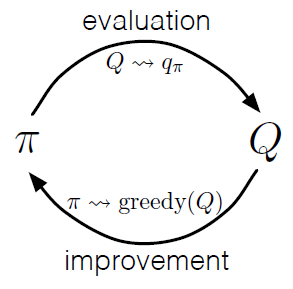1. 利用当前的值函数$\pi(s) = \arg\max_{a \in \mathcal{A}} Q(s, a)$贪心地更新策略
2. 使用新的策略$\pi$(例如$\epsilon-\text{greedy}$来进行探索-利用的平衡)生成一个新的 episode.
3. 使用新的 episode 估计新的 Q值: $q_\pi(s, a) = \frac{\sum_{t=1}^T \big( \mathbb{I}[S_t = s, A_t = a] \sum_{k=0}^{T-t-1} \gamma^k R_{t+k+1} \big)}{\sum_{t=1}^T \mathbb{I}[S_t = s, A_t = a]}$

First-visit 的 MC 方法: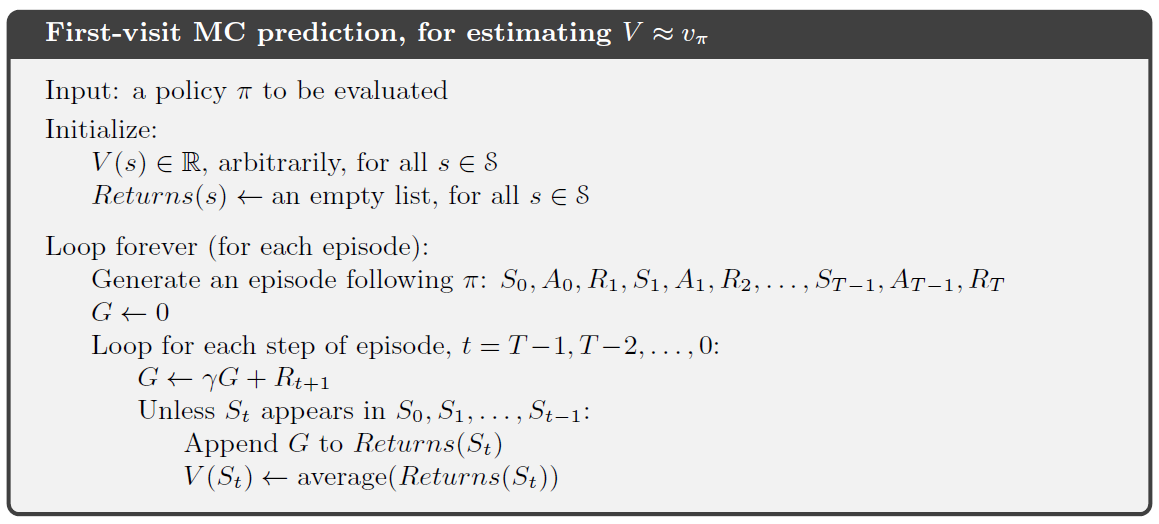## 时间差分学习(Temporal-Difference Learning)

### 自助法(Bootstrapping)

TD 学习估计当前状态的价值使用了其他状态的价值.What exactly is bootstrapping in reinforcement learning?

### 值估计(Value Estimation)

TD 学习的关键思想是使用估计的 return: $R_{t+1}+\gamma{V}(S_{t+1})$, 即 TD 目标(TD target)来更新值函数$V(S_t)$. 参数$\alpha$用来更新值函数更新的步幅:

\begin{aligned} V(S_t) &\leftarrow (1- \alpha) V(S_t) + \alpha G_t \\ V(S_t) &\leftarrow V(S_t) + \alpha (G_t - V(S_t)) \\ V(S_t) &\leftarrow V(S_t) + \alpha (R_{t+1} + \gamma V(S_{t+1}) - V(S_t)) \end{aligned}

$$Q(S_t, A_t) \leftarrow Q(S_t, A_t) + \alpha (R_{t+1} + \gamma Q(S_{t+1}, A_{t+1}) - Q(S_t, A_t))$$

### SARSA: On-policy TD 控制

SARSA 可以指的是用于更新Q-值的序列: $\dots, S_t, A_t, R_{t+1}, S_{t+1}, A_{t+1}, \dots$. 与 GPI算法相似:

1. 在时间$t$, 从状态$S_t$开始, 根据 Q-值选择行为$A_t=\arg\max_{a\in\mathcal{A}}Q(S_t,a)$; 通常可用 $\epsilon-\text{greddy}$策略.
2. 采取动作$A_t$, 收到即时回报$R_{t+1}$和下一个状态$S_{t+1}$
3. 按照同样的策略选择下一个动作: $A_{t+1} = \arg\max_{a \in \mathcal{A}} Q(S_{t+1}, a)$
4. 更新 action-value 函数值: $Q(S_t, A_t) \leftarrow Q(S_t, A_t) + \alpha (R_{t+1} + \gamma Q(S_{t+1}, A_{t+1}) - Q(S_t, A_t))$
5. $t=t+1$ 回到步骤1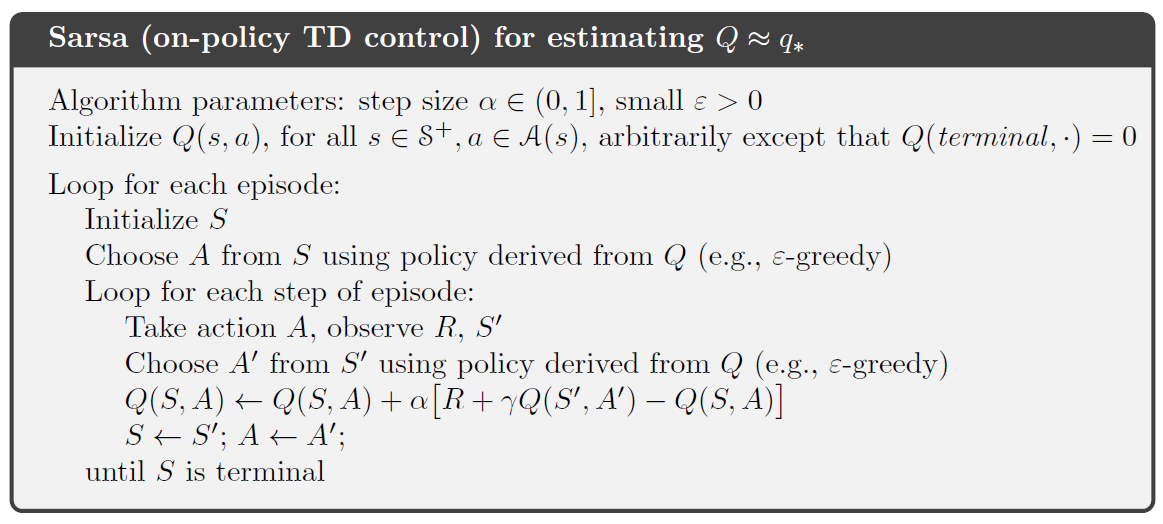### Q-Learning: Off-policy TD 控制

1. 在时间$t$, 从状态$S_t$开始, 根据 Q-值选择行为$A_t=\arg\max_{a\in\mathcal{A}}Q(S_t,a)$; 通常可用 $\epsilon-\text{greddy}$策略.
2. 采取动作$A_t$, 收到即时回报$R_{t+1}$和下一个状态$S_{t+1}$
3. 更新 action-value 函数值: $Q(S_t, A_t) \leftarrow Q(S_t, A_t) + \alpha (R_{t+1} + \gamma Q(S_{t+1}, A_{t+1}) - Q(S_t, A_t))$
4. $t=t+1$ 回到步骤1
5. 详细算法步骤: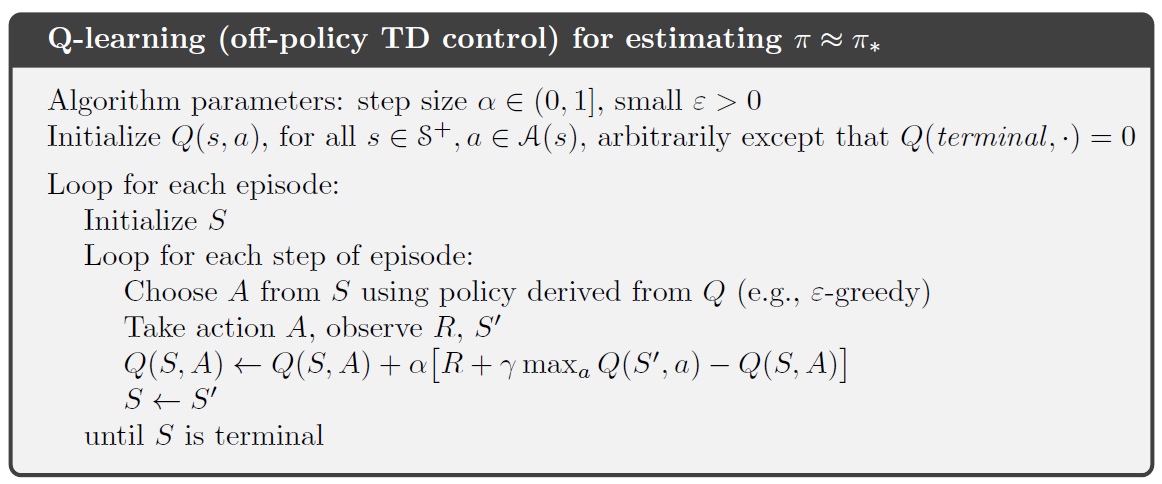Q-leaning 的 backup 方式与 SARSA 不同: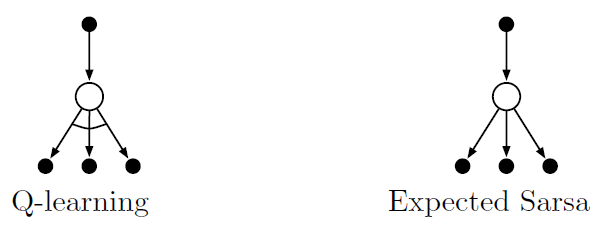## TD 和 MC 结合的学习

n $G_t$ 备注
$n=1$ $G_t^{(1)} = R_{t+1} + \gamma V(S_{t+1})$ TD 学习
$n=2$ $G_t^{(2)} = R_{t+1} + \gamma R_{t+2} + \gamma^2 V(S_{t+2})$
$\cdots$
$n=n$ $G_t^{(n)} = R_{t+1} + \gamma R_{t+2} + \dots + \gamma^{n-1} R_{t+n} + \gamma^n V(S_{t+n})$
$\cdots$
$n=\infty$ $G_t^{(\infty)} = R_{t+1} + \gamma R_{t+2} + \dots + \gamma^{T-t-1} R_T + \gamma^{T-t} V(S_T)$ MC 估计

$$V(S_t) \leftarrow V(S_t) + \alpha (G_t^{(n)} - V(S_t))$$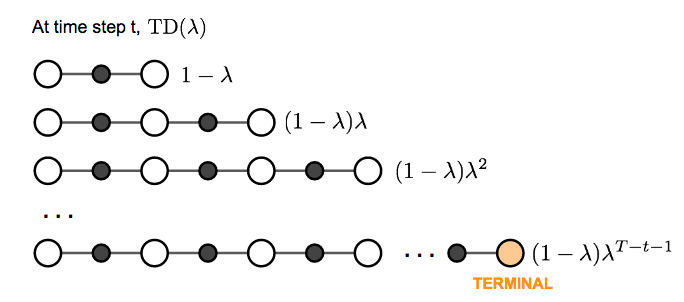\begin{aligned} \text{let } S &= 1 + \lambda + \lambda^2 + \dots \\ S &= 1 + \lambda(1 + \lambda + \lambda^2 + \dots) \\ S &= 1 + \lambda S \\ S &= 1 / (1-\lambda) \end{aligned}